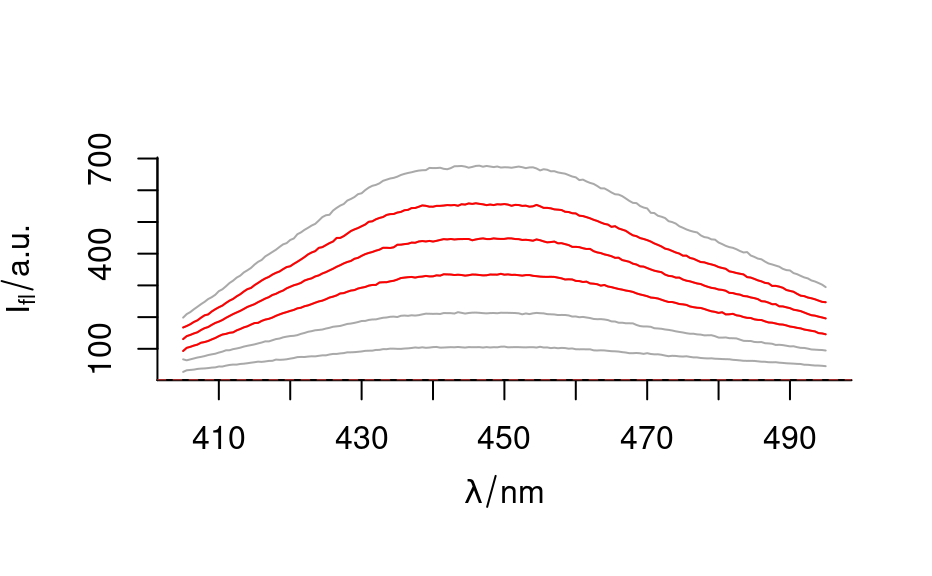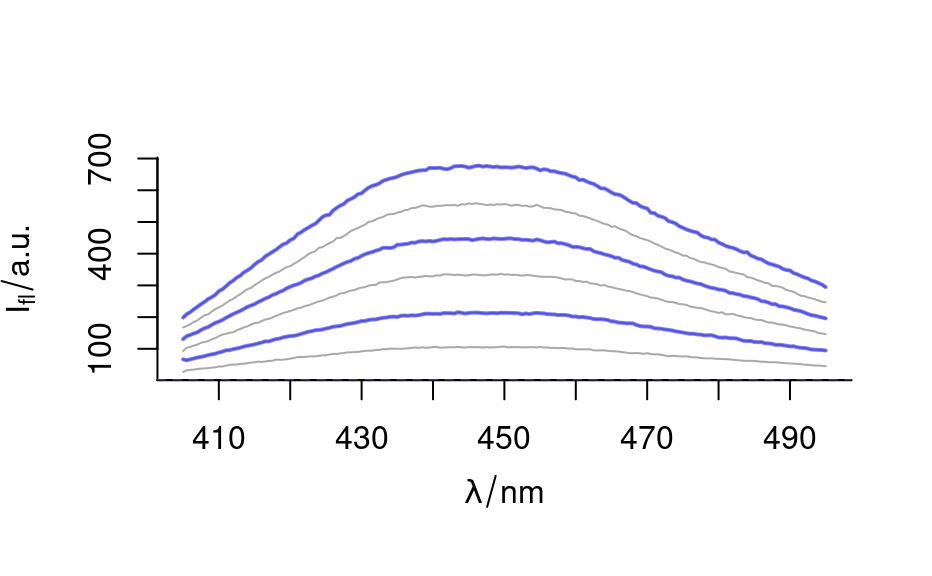sample() takes a random sample (drawn with or without replacement) of rows from the object x.

# S4 method for hyperSpec
sample(x, size, replace = FALSE, prob = NULL, index = FALSE)

# S4 method for data.frame
sample(x, size, replace = FALSE, prob = NULL, index = FALSE, drop = FALSE)

# S4 method for matrix
sample(x, size, replace = FALSE, prob = NULL, index = FALSE, drop = FALSE)

## Arguments

x

hyperSpec object, data.frame or matrix to sample rows from.

size

(integer): Positive integer giving the number of spectra (rows) to choose. If missing, defaults to the number of rows in the object: size = nrow(x).

replace

(logical): Should sampling be with replacement?

prob

(numeric): A vector of probability weights for obtaining the elements of the vector being sampled.

index

(logical): If FALSE, object of class(x) is returned, if TRUE, numeric vector is returned.

drop

(logical): See base::drop(), by default, do not drop dimensions of the result. Applicable only if index = FALSE.

## Value

• If index = FALSE, function returns a hyperSpec object, data.frame or matrix (object of the same class as x) with size rows.

• If index = TRUE, function returns a vector with row indices of size size suitable for subsetting rows of x.

base::sample()

## Author

C. Beleites, V. Gegzna

## Examples

set.seed(2021)

sample(flu, 3)
#> hyperSpec object
#>    3 spectra
#>    3 data columns
#>    181 data points / spectrum

sample(flu, 3, index = TRUE)
#>  4 6 2

sample(flu, 3, replace = TRUE, index = TRUE)
#>  6 3 6

sample(flu, 8, replace = TRUE, index = TRUE)
#>  6 5 1 4 3 4 2 3

plot(flu, col = "darkgray")
plot(sample(flu, 3), col = "red", add = TRUE)plot(flu, col = "darkgray")
plot(sample(flu, 3, replace = TRUE),
col = "#0000FF80", add = TRUE,
lines.args = list(lwd = 2)
)sample(cars, 2)
#>    speed dist
#> 37    19   46
#> 22    14   60

sample(cars, 2, index = TRUE)
#>  31 48

sample(matrix(1:24, 6), 2)
#>      [,1] [,2] [,3] [,4]
#> [1,]    2    8   14   20
#> [2,]    3    9   15   21

sample(matrix(1:24, 6), 2, index = TRUE)
#>  4 5